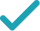Type a math problemSolve for x, ySteps Using Substitution
Steps Using Matrices
Steps Using Elimination
Steps Using Substitution
Consider the first equation. Multiply both sides of the equation by , the least common multiple of .
Consider the second equation. Multiply both sides of the equation by , the least common multiple of .
To solve a pair of equations using substitution, first solve one of the equations for one of the variables. Then substitute the result for that variable in the other equation.
Choose one of the equations and solve it for by isolating on the left hand side of the equal sign.
Add to both sides of the equation.
Divide both sides by .
Multiply times .
Substitute for in the other equation, .
Multiply times .
Subtract from both sides of the equation.
Divide both sides of the equation by , which is the same as multiplying both sides by the reciprocal of the fraction.
Substitute for in . Because the resulting equation contains only one variable, you can solve for directly.
Multiply times .
The system is now solved.Giving is as easy as 1, 2, 3
Get 1,000 points to donate to a school of your choice when you join Give With Bing
2x-3y=48
Consider the first equation. Multiply both sides of the equation by 6, the least common multiple of 3,2.
3x+5y=15
Consider the second equation. Multiply both sides of the equation by 15, the least common multiple of 5,3.
2x-3y=48,3x+5y=15
To solve a pair of equations using substitution, first solve one of the equations for one of the variables. Then substitute the result for that variable in the other equation.
2x-3y=48
Choose one of the equations and solve it for x by isolating x on the left hand side of the equal sign.
2x=3y+48
Add 3y to both sides of the equation.
x=\frac{1}{2}\left(3y+48\right)
Divide both sides by 2.
x=\frac{3}{2}y+24
Multiply \frac{1}{2}=0.5 times 48+3y.
3\left(\frac{3}{2}y+24\right)+5y=15
Substitute \frac{3y}{2}+24 for x in the other equation, 3x+5y=15.
\frac{9}{2}y+72+5y=15
Multiply 3 times \frac{3y}{2}+24.
\frac{19}{2}y+72=15
\frac{19}{2}y=-57
Subtract 72 from both sides of the equation.
y=-6
Divide both sides of the equation by \frac{19}{2}=9.5, which is the same as multiplying both sides by the reciprocal of the fraction.
x=\frac{3}{2}\left(-6\right)+24
Substitute -6 for y in x=\frac{3}{2}y+24. Because the resulting equation contains only one variable, you can solve for x directly.
x=-9+24
Multiply \frac{3}{2}=1.5 times -6.
x=15
x=15,y=-6
The system is now solved.
2x-3y=48
Consider the first equation. Multiply both sides of the equation by 6, the least common multiple of 3,2.
3x+5y=15
Consider the second equation. Multiply both sides of the equation by 15, the least common multiple of 5,3.
2x-3y=48,3x+5y=15
Put the equations in standard form and then use matrices to solve the system of equations.
\left(\begin{matrix}2&-3\\3&5\end{matrix}\right)\left(\begin{matrix}x\\y\end{matrix}\right)=\left(\begin{matrix}48\\15\end{matrix}\right)
Write the equations in matrix form.
inverse(\left(\begin{matrix}2&-3\\3&5\end{matrix}\right))\left(\begin{matrix}2&-3\\3&5\end{matrix}\right)\left(\begin{matrix}x\\y\end{matrix}\right)=inverse(\left(\begin{matrix}2&-3\\3&5\end{matrix}\right))\left(\begin{matrix}48\\15\end{matrix}\right)
Left multiply the equation by the inverse matrix of \left(\begin{matrix}2&-3\\3&5\end{matrix}\right).
\left(\begin{matrix}1&0\\0&1\end{matrix}\right)\left(\begin{matrix}x\\y\end{matrix}\right)=inverse(\left(\begin{matrix}2&-3\\3&5\end{matrix}\right))\left(\begin{matrix}48\\15\end{matrix}\right)
The product of a matrix and its inverse is the identity matrix.
\left(\begin{matrix}x\\y\end{matrix}\right)=inverse(\left(\begin{matrix}2&-3\\3&5\end{matrix}\right))\left(\begin{matrix}48\\15\end{matrix}\right)
Multiply the matrices on the left hand side of the equal sign.
\left(\begin{matrix}x\\y\end{matrix}\right)=\left(\begin{matrix}\frac{5}{2\times 5-\left(-3\times 3\right)}&-\frac{-3}{2\times 5-\left(-3\times 3\right)}\\-\frac{3}{2\times 5-\left(-3\times 3\right)}&\frac{2}{2\times 5-\left(-3\times 3\right)}\end{matrix}\right)\left(\begin{matrix}48\\15\end{matrix}\right)
For the 2\times 2 matrix \left(\begin{matrix}a&b\\c&d\end{matrix}\right), the inverse matrix is \left(\begin{matrix}\frac{d}{ad-bc}&\frac{-b}{ad-bc}\\\frac{-c}{ad-bc}&\frac{a}{ad-bc}\end{matrix}\right), so the matrix equation can be rewritten as a matrix multiplication problem.
\left(\begin{matrix}x\\y\end{matrix}\right)=\left(\begin{matrix}\frac{5}{19}&\frac{3}{19}\\-\frac{3}{19}&\frac{2}{19}\end{matrix}\right)\left(\begin{matrix}48\\15\end{matrix}\right)
Do the arithmetic.
\left(\begin{matrix}x\\y\end{matrix}\right)=\left(\begin{matrix}\frac{5}{19}\times 48+\frac{3}{19}\times 15\\-\frac{3}{19}\times 48+\frac{2}{19}\times 15\end{matrix}\right)
Multiply the matrices.
\left(\begin{matrix}x\\y\end{matrix}\right)=\left(\begin{matrix}15\\-6\end{matrix}\right)
Do the arithmetic.
x=15,y=-6
Extract the matrix elements x and y.
2x-3y=48
Consider the first equation. Multiply both sides of the equation by 6, the least common multiple of 3,2.
3x+5y=15
Consider the second equation. Multiply both sides of the equation by 15, the least common multiple of 5,3.
2x-3y=48,3x+5y=15
In order to solve by elimination, coefficients of one of the variables must be the same in both equations so that the variable will cancel out when one equation is subtracted from the other.
3\times 2x+3\left(-3\right)y=3\times 48,2\times 3x+2\times 5y=2\times 15
To make 2x and 3x equal, multiply all terms on each side of the first equation by 3 and all terms on each side of the second by 2.
6x-9y=144,6x+10y=30
Simplify.
6x-6x-9y-10y=144-30
Subtract 6x+10y=30 from 6x-9y=144 by subtracting like terms on each side of the equal sign.
-9y-10y=144-30
Add 6x to -6x. Terms 6x and -6x cancel out, leaving an equation with only one variable that can be solved.
-19y=144-30
-19y=114